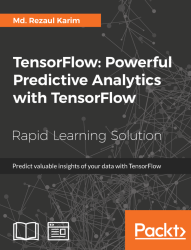•#### TensorFlow: Powerful Predictive Analytics with TensorFlow#### Overview of this book

Predictive analytics discovers hidden patterns from structured and unstructured data for automated decision making in business intelligence. Predictive decisions are becoming a huge trend worldwide, catering to wide industry sectors by predicting which decisions are more likely to give maximum results. TensorFlow, Google’s brainchild, is immensely popular and extensively used for predictive analysis. This book is a quick learning guide on all the three types of machine learning, that is, supervised, unsupervised, and reinforcement learning with TensorFlow. This book will teach you predictive analytics for high-dimensional and sequence data. In particular, you will learn the linear regression model for regression analysis. You will also learn how to use regression for predicting continuous values. You will learn supervised learning algorithms for predictive analytics. You will explore unsupervised learning and clustering using K-meansYou will then learn how to predict neighborhoods using K-means, and then, see another example of clustering audio clips based on their audio features. This book is ideal for developers, data analysts, machine learning practitioners, and deep learning enthusiasts who want to build powerful, robust, and accurate predictive models with the power of TensorFlow. This book is embedded with useful assessments that will help you revise the concepts you have learned in this book. This book is repurposed for this specific learning experience from material from Packt's Predictive Analytics with TensorFlow by Md. Rezaul Karim.
TensorFlow: Powerful Predictive Analytics with TensorFlowCreditsPrefaceFree Chapter
From Data to Decisions – Getting Started with TensorFlowPutting Data in Place – Supervised Learning for Predictive AnalyticsClustering Your Data – Unsupervised Learning for Predictive AnalyticsUsing Reinforcement Learning for Predictive Analytics## Summary

TensorFlow is designed to make the predictive analytics through the machine and deep learning easy for everyone, but using it does require understanding some general principles and algorithms. Furthermore, the latest release of TensorFlow comes with lots of exciting features. Thus I also tried to cover them so that you can use them with ease. I have shown how to install TensorFlow on different platforms including Linux, Windows, and Mac OS. In summary, here is a brief recap of the key concepts of TensorFlow explained in this lesson:

• Graph: each TensorFlow computation can be represented as a set of dataflow graphs where each graph is built as a set of operation objects. There are three core graph data structures:

1. `tf.Graph`

2. `tf.Operation`

3. `tf.Tensor`

• Operation: A graph node takes tensors as input and also produces a tensor as output. A node can be represented by an operation object for performing units of computations such as addition, multiplication, division, subtraction or more complex operation.

• Tensor: Tensors are like high-dimensional array objects. In other words, they can be represented as edges of a dataflow graph but still they don't hold any value produced out of an operations.

• Session: A session object is an entity that encapsulates the environment in which operation objects are executed for running calculations on the dataflow graph. As a result, the tensors objects are evaluated inside the `run()` or `eval()` invocation.

In a later section of the lesson, we introduced TensorBoard, which is a powerful tool for analyzing and debugging neural network models, the lesson ended with an example that shows how to implement a simple neuron model and how to analyze its learning phase with TensorBoard.

Predictive models often perform calculations during live transactions, for example, to evaluate the risk or opportunity of a given customer or transaction, in order to guide a decision. With advancements in computing speed, individual agent modeling systems have become capable of simulating human behavior or reactions to given stimuli or scenarios.

In the next lesson, we will cover linear models for regression, classification, and clustering and dimensionality reduction and will also give some insights about some performance measures.